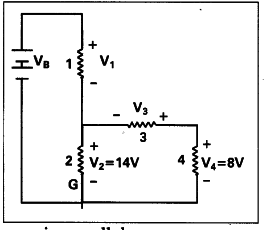# Observe the circuit and answer the questions given below

Observe the circuit and answer the questions given below.
i) Are resistors 3 and 4 in series ?
ii) Are resistors 1 and 2 in series ?
iii) Is the battery in series with any resistor ?
iv) What is the potential drop across the resistor 3 ?
v) What is total emf in the circuit if the potential drop across resistor 1 is 6V?1. No. Resistors 3 and 4 are not in series. They are in parallel.
2. Yes. The resistors 1 and 2 are in series.
3. No.
4. As resistors 3 and 4 are in parallel, same potential difference will be allowed through them. Hence the potential drop across resistor 3 is 8V
5. Total emf = V1 + V2 + V3 + V4 = 6V + 14V + 8V +8V= 36V